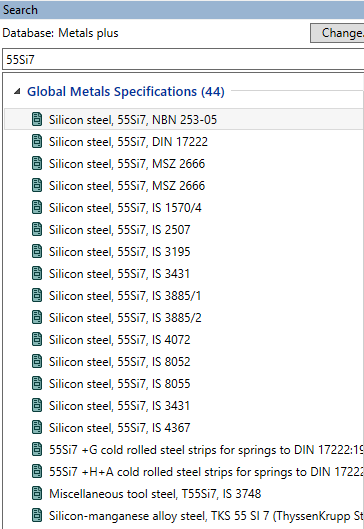## Materials

Topics relate to Granta Design and more

•khokon_sazzal
Subscriber
Hi, I am simulating fatigue loading on a spring steel (55si7 or AISI 55S7) specimen in Ansys. But due to proper material properties, specially S-N curve, I am unable to do it.n
•Govindan Nagappan
Ansys Employee
@khokon_sazzal nIf you have access to the Granta Selector product, you can search the database for the material properties. I do see several different materials show up on a quick searchnIf you do not have access to Granta, you may have to check with your supplier for the material propertiesn
•Daniel Shaw
Ansys Employee
Obtaining S-N curves can be difficult. Ansys provides some in Mechanical and some in Granta. Otherwise, the user must obtain them. You may need to contact a material supplier to to obtain them.n
•ramgopisetti
Subscriber
You can construct an therotical SN curve if you have the Ultimate tensile strength of your material. the ordinates of that curve will be as followsnFor an four point curve( mostly helpful)nat 0 Cycle = SUTnat 1E3 Cycle = n x SUT where n =0.8 and changes based on Manufacturing ( refer to shigley textbook)nat 1E6 Cycle = 0.5 x SUT for SUT <= 1400MPa or 700 MPa if less than 1400MPa nand at infinte cucle it just continues .n use the followign python script to visulise the plot nimport matplotlib.pyplot as pltnimport numpy as npnfrom scipy.interpolate import interp1dnSUT=650nSYT=510nSE=118.4ndef sn(SUT,SYT,R,a,m):n plt.subplot(2, 1, 1)n X=np.array([0,1E3, 1E6,1E7] )n Y=np.array([SUT, 0.8*SUT, 0.5*SUT, 0.5*SUT])n plt.xlabel(Cycles)n plt.ylabel(Strength (MPa))n plt.grid()n plt.plot(X,Y)n plt.subplot(2, 1, 2)n X1 = np.array([SYT, 0])n X2 = np.array([SUT, 0])n Y1 = np.array([0, SYT])n Y2 = np.array([0, 0.5*SUT])n plt.plot(X1, Y1, label='Yield Line')n plt.plot(X2, Y2, label='Modified GoodMan')n yu = R * X2n plt.plot(X2, yu, label='Ratio=%f' % (R))n plt.axis([0, SUT, 0, SYT])n plt.hlines(y=a,xmin=0,xmax=m)n plt.vlines(x=m,ymin=0,ymax=a)n plt.xlabel(Mean stress (MPa))n plt.ylabel(Alternating Stress (MPa))n plt.legend(loc='upper right')nn plt.show()nThis is theroy,you can extend this idea as you want and fit your results.
•David Mercier
Ansys Employee
Just to add a comment about material data in Granta Selector. You can have access to S-N curves, at least to fatigue strength model like given below for a low alloy steel, in the database MaterialUniverse which gives access to generic material datasheets. In such datasheet, the fatigue stregth model, displayed as an S-N curve on the datasheet, shows the highest cyclic stress amplitude the material can survive, when the stress is repeatedly applied and relaxed (or reversed) for a given number of cycles.nnn